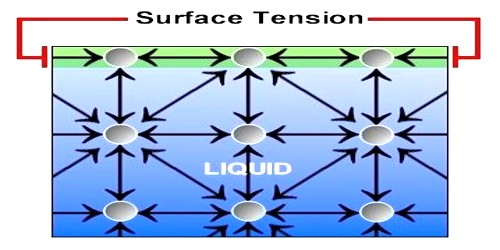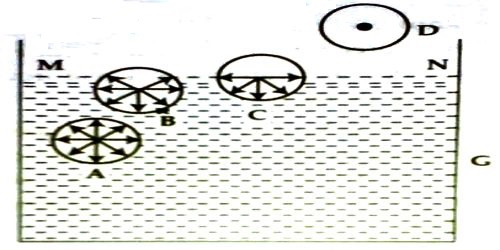# Explanation of Surface Tension by Laplace’s Molecular Theory

Explanation of Surface Tension by Laplace’s molecular theory of surface tension

To explain the phenomenon of surface tension Laplace gave a molecular theory which is known as Laplace’s molecular theory of surface tension.

Let consider four molecules A, B, C and D of a liquid [Figure]. Sphere A is well inside this liquid: B. near the surface of the liquid; C, just on the surface and D, above the liquid surface. Let us draw a sphere of influence around them. Since the sphere of influence of molecule A is fully inside the liquid, it is attracted equally in all directions by other molecules. So, the resultant cohesive force on it is zero. So, it will remain in a state as it was.Since the shpere of influence of molecule B lies partly outside the liquid, this part contains only a few molecules of vapour compared to the liquid molecules in the lower part. So, the downward attractive force due to liquid molecules exceeds that of upward force of attraction by vapour molecules. So, there S a resultant downward force acting on B.

Since the molecule C lies on the surface of the liquid the one-half of its sphere of influence lies inside and the other half lies above the surface of the liquid. This part contains only few molecules of gas or vapour. But the entire lower half contains liquid molecules and thus the downward force, in this case is maximum. This phenomenon will be observed for all the molecules lying on the surface. This downward or inward force per unit area of a liquid surface is called cohesive pressure, which is the cause of cohesion.

The molecule D lies outside the surface of the liquid. The sphere of influence is outside the liquid, so the downward force due to liquid molecules is zero. So, the molecule moves freely, like gas molecules. It is thus clear that all over the surface of the liquid MN there is a downward pull due to attraction between molecules. This surface tends to contract, i.e., it tends to decrease the area of surface MN. Surface tension originates from this tendency of surface contraction. This tension acts tangentially to the surface of the liquid.

This is the molecular explanation of surface tension according to Laplace.